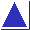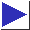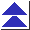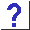# comp.lang.c FAQ list ·Question 14.5

Q: What's a good way to check for ``close enough'' floating-point equality?

A: Since the absolute accuracy of floating point values varies, by definition, with their magnitude, the best way of comparing two floating point values is to use an accuracy threshold which is relative to the magnitude of the numbers being compared. Rather than

```	double a, b;
...
if(a == b)	/* WRONG */
```
use something like
```	#include <math.h>

if(fabs(a - b) <= epsilon * fabs(a))
```
where epsilon is a value chosen to set the degree of ``closeness'' (and where you know that a will not be zero). The precise value of epsilon may still have to be chosen with care: its appropriate value may be quite small and related only to the machine's floating-point precision, or it may be larger if the numbers being compared are inherently less accurate or are the results of a chain of calculations which compounds accuracy losses over several steps. (Also, you may have to make the threshold a function of b, or of both a and b.)

A decidedly inferior approach, not generally recommended, would be to use an absolute threshold:

```	if(fabs(a - b) < 0.001)		/* POOR */
```
Absolute ``fuzz factors'' like 0.001 never seem to work for very long, however. As the numbers being compared change, it's likely that two small numbers that should be taken as different happen to be within 0.001 of each other, or that two large numbers, which should have been treated as equal, differ by more than 0.001 . (And, of course, the problems merely shift around, and do not go away, when the fuzz factor is tweaked to 0.005, or 0.0001, or any other absolute number.)

Doug Gwyn suggests using the following ``relative difference'' function. It returns the relative difference of two real numbers: 0.0 if they are exactly the same, otherwise the ratio of the difference to the larger of the two.

```#define Abs(x)    ((x) < 0 ? -(x) : (x))
#define Max(a, b) ((a) > (b) ? (a) : (b))

double RelDif(double a, double b)
{
double c = Abs(a);
double d = Abs(b);

d = Max(c, d);

return d == 0.0 ? 0.0 : Abs(a - b) / d;
}
```
Typical usage is
```	if(RelDif(a, b) <= TOLERANCE) ...
```

References: Knuth Sec. 4.2.2 pp. 217-8